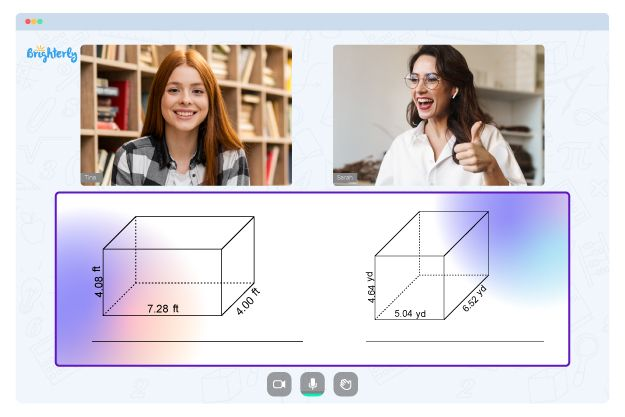# Parallel Lines and Transversals Worksheets

Lines that are always the same distance apart and in the same plane are called parallel lines. Those that cross two or more other lines at different points are called transversals. In geometry, parallel lines and transversals come up a lot.

Parallel lines and transversals worksheet answer key is an increasingly popular tool that can help students practice and comprehend properties of angles and solve real-world problems. This article will discuss worksheet 3 parallel lines cut by a transversal and the ways it can help students understand these ideas.

## Parallel Lines Worksheets

The parallel lines worksheets are intended to assist students in comprehending the properties of parallel lines. The exercises and problems on these worksheets typically require students to use their understanding of parallel lines to solve them. Students might be required to identify parallel lines in a diagram or use parallel lines to solve a geometric problem, for instance.

These worksheets may also include drag-and-drop exercises or other interactive activities that give students a hands-on opportunity to check out the properties of parallel lines.

## Transversals Lines Worksheets

The purpose of transversal worksheets is to help students learn about and practice working with transversal lines. Many problems on transversal worksheets involve figuring out, measuring, and classifying angles. Identifying corresponding, alternate interior, and alternate exterior angles might be some of these issues.

Transversal worksheets help teach students about transversals and allow them to practice working with them. The worksheets may also include problems requiring a protractor to measure angles. You can recommend worksheets on parallel lines and transversals alongside other resources like books and videos to give students a complete understanding of these ideas.

Math for Kids

Is Your Child Struggling With Math?
1:1 Online Math Tutoring## Benefits of Parallel Lines Cut by a Transversal Worksheet

Students can learn about angles formed by parallel lines cut by a transversal using parallel lines and transversal worksheets. The proving lines parallel worksheet will help students solve geometry problems. They can also use the tools for self-study.

Students use these worksheets to review and practice the concepts they have learned in class and to prepare for tests and quizzes. The resources improve their ability to solve problems involving parallel lines and transversals by working through worksheets on the topics of parallel lines and transversals.

A variation of these tools – parallel lines cut by a transversal worksheet answers – is also making the rounds. The interactive activities and hands-on practice on these worksheets help students comprehend the properties of parallel lines and transversals. The parallel lines and transversals worksheet answers will let students know when their solutions miss the mark and how to retrace their steps.

They can use the knowledge on the angles created by transversals and parallel lines to create and solve equations for missing angles.### Parallel Lines and Transversals Worksheets PDF

Parallel Lines And Transversals Worksheet Answers### Parallel Lines and Transversals Worksheets PDF

Proving Lines Parallel Worksheet### Parallel Lines and Transversals Worksheets PDF

Parallel Lines Cut By A Transversal Worksheet### Parallel Lines and Transversals Worksheets PDF

Parallel Lines And Transversals Worksheet

Problems with Geometry?• Start learning with an online tutor.

Is your child finding it challenging to grasp of geometry? An online tutor could be the solution.